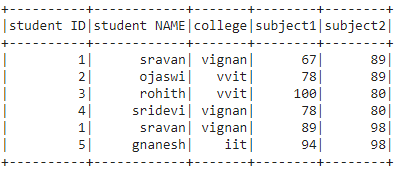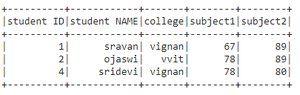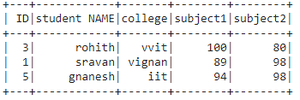# How to select a range of rows from a dataframe in PySpark ?

• Last Updated : 29 Jun, 2021

In this article, we are going to select a range of rows from a PySpark dataframe.

It can be done in these ways:

Attention geek! Strengthen your foundations with the Python Programming Foundation Course and learn the basics.

To begin with, your interview preparations Enhance your Data Structures concepts with the Python DS Course. And to begin with your Machine Learning Journey, join the Machine Learning - Basic Level Course

• Using filter().
• Using where().
• Using SQL expression.

Creating Dataframe for demonstration:

## Python3

 `# importing module``import` `pyspark`` ` `# importing sparksession from pyspark.sql module``from` `pyspark.sql ``import` `SparkSession`` ` `# creating sparksession and giving an app name``spark ``=` `SparkSession.builder.appName(``'sparkdf'``).getOrCreate()`` ` `# list  of students  data``data ``=` `[[``"1"``, ``"sravan"``, ``"vignan"``, ``67``, ``89``],``        ``[``"2"``, ``"ojaswi"``, ``"vvit"``, ``78``, ``89``],``        ``[``"3"``, ``"rohith"``, ``"vvit"``, ``100``, ``80``],``        ``[``"4"``, ``"sridevi"``, ``"vignan"``, ``78``, ``80``],``        ``[``"1"``, ``"sravan"``, ``"vignan"``, ``89``, ``98``],``        ``[``"5"``, ``"gnanesh"``, ``"iit"``, ``94``, ``98``]]`` ` `# specify column names``columns ``=` `[``'student ID'``, ``'student NAME'``,``           ``'college'``, ``'subject1'``, ``'subject2'``]`` ` `# creating a dataframe from the lists of data``dataframe ``=` `spark.createDataFrame(data, columns)`` ` `# display dataframe``dataframe.show()`

Output:Method 1: Using filter()

This function is used to filter the dataframe by selecting the records based on the given condition.

Syntax: dataframe.filter(condition)

Example: Python code to select the dataframe based on subject2 column.

## Python3

 `# select dataframe between``# 23 and 78 marks in subject2 ``dataframe.``filter``(``  ``dataframe.subject1.between(``23``,``78``)).show()`

Output:Method 2: Using where()

This function is used to filter the dataframe by selecting the records based on the given condition.

Syntax: dataframe.where(condition)

Example 1: Python program to select dataframe based on subject1 column.

## Python3

 `# select dataframe between``# 85 and 100 in subject1 column``dataframe.``filter``(``  ``dataframe.subject1.between(``85``,``100``)).show()`

Output:Example 2: Select rows in dataframe by college column

## Python3

 `# select dataframe in college column ``# for vvit``dataframe.``filter``(``  ``dataframe.college.between(``"vvit"``,``"vvit"``)).collect()`

Output:

[Row(ID=’2′, student NAME=’ojaswi’, college=’vvit’, subject1=78, subject2=89),

Row(ID=’3′, student NAME=’rohith’, college=’vvit’, subject1=100, subject2=80)]

Method 3: Using SQL Expression

By using SQL query with between() operator we can get the range of rows.

Syntax: spark.sql(“SELECT * FROM my_view WHERE column_name between value1 and value2”)

Example 1: Python program to select rows from dataframe based on subject2 column

## Python3

 `# create view for the dataframe``dataframe.createOrReplaceTempView(``"my_view"``)`` ` `# data subject1 between 23 and 78``spark.sql("SELECT ``*` `FROM my_view WHERE\``subject1 between ``23` `and` `78``").collect()`

Output:

[Row(student ID=’1′, student NAME=’sravan’, college=’vignan’, subject1=67, subject2=89),

Row(student ID=’2′, student NAME=’ojaswi’, college=’vvit’, subject1=78, subject2=89),

Row(student ID=’4′, student NAME=’sridevi’, college=’vignan’, subject1=78, subject2=80)]

Example 2: Select based on ID

## Python3

 `# create view for the dataframe``dataframe.createOrReplaceTempView(``"my_view"``)`` ` `# data subject1 between 23 and 78``spark.sql("SELECT ``*` `FROM my_view WHERE\``ID` `between ``1` `and` `3``").collect()`

Output:

[Row(ID=’1′, student NAME=’sravan’, college=’vignan’, subject1=67, subject2=89),

Row(ID=’2′, student NAME=’ojaswi’, college=’vvit’, subject1=78, subject2=89),

Row(ID=’3′, student NAME=’rohith’, college=’vvit’, subject1=100, subject2=80),

Row(ID=’1′, student NAME=’sravan’, college=’vignan’, subject1=89, subject2=98)]

My Personal Notes arrow_drop_up